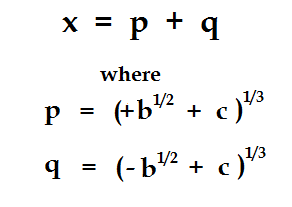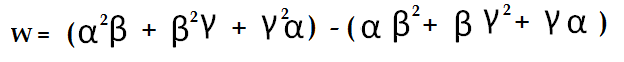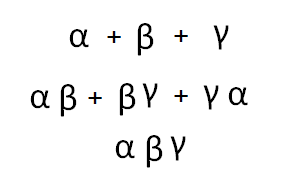## Tuesday, June 19, 2012

### The Fifth Degree: Part III

When we left off, I had shown an expression in rational numbers b and c which I claimed gave us three unique values for the solutions of a cubic equation. This would be a small miracle because by the time you've taken square roots of this and cube roots of that, you have at least six possible values for the simplest possible expression. In fact, the expression I gave ought to have had nine possible values:You choose one of three values from column A, and one of three values from column B. Nine values for x. How is that the solution of a cubic?

It's not. It's the solution of a ninth-degree equation. But...and here's the catch...sometimes, the ninth degree reduces to a cubic. If c-squared take-away b happens to be a perfect cube....look what happens. For example, if c is 5 and b is -2, then the expression pq, as usual, takes on nine possible values. But three of those values come out to pq = 3. (The other six values are equal to 3w, where w is a cube root of unity.) What it means is that while all three p's are indistinguishable from each other, and all three q's are also indistinguishable, not all three pairs of p and q are indistinguishable. We choose only those pairs p and q whose product is a rational number. And those pairs give us three unique values which are the solution of a cubic equation.

It's fun to work it back from the other direction. Let the three solutions of our cubic equation be alpha, beta, and gamma. It's not too hard to show that linear combinations of all polynomials in alpha, beta, and gamma up to second degree in any variable form an algebraically closed field. (Obviously you don't have to consider third powers of alpha etc. because these always reduce to expressions in lower powers using the cubic equation itself. In particular, we can identify one particular element of this algebraic field which we will see is the solution of a quadratic equation. Take a deep breath, because here goes:(CORRECTION: When I first posted this, I inadvertently squared one of the bracketed terms.) In fact, w is not just the solution of a quadratic equation, its the square root of a rational number. There are two ways we can see this. First, we can square it out and examine the terms. But we don't have to work quite that hard. Remember, every symmetric polynomial in alpha, beta, and gamma is a rational numbers. This property is inherited from the elementary symmetric polynomails:You should recognize these as the rational coefficients of the cubic equation. So they are all just numbers. And it's not hard to show that any symmetric combination of alpha, beta, and gamma can be expressed as a function of these three elementary building blocks. So if it's symmetric, it's a rational number.

Now, the expression we built for w is not quite symmetric, but it's not far from it. If we take the square of w, we get six symmetric terms in alpha-squared-beta etc, and then we get a bunch of cross terms which, when the dust has all settled, turn out to be symmetric as well. So w-squared is rational.

There's another way we could have seen this by looking at the group theoretic  properties of the field. For a general cubic equation, you can take any permutation of the three roots and create a new field identical to the original field, but with the numbers simply shuffled around. Except for one thing: you can't shuffle around the rational numbers. Everything else is fair game. It's the logical consequence of alpha, beta and gamma being indistinuishable from one another. If I swap alpha and beta, everything I do with them algebraically has to have the same ultimate result at the point where I write a formula that evaluates to a rational value. I'm not allowed to complain that this irrational number is different from that irrational number, because the only properties that really count are those that lead to rational numbers. So I can swap alpha and beta, leaving gamma fixed. I can shift alpha to beta, beta to alpha, and alpha to gamma. The irrational numbers all shuffle themselves accordingly, but the rationals stay fixed.

But what about our w, the expression I said was a square root? If you look at it carefully, you should see that if we switch alpha and beta, it simply reverses the sign of w. If we switch beta and gamma....same thing. If we cycle alpha to beta, beta to gamma, and gamma to alpha....we just get w itself, without even a change of sign.

What kind of number is algebraically indistinguishalbe from its own negative? Why, the square root of a number. The positive square root of two is indistinguishable from the negative square root of two. The field which you get by adjoining the square root of two to the rationals is a field with only one homomorphism of itself...the homomophism generated by swapping the positive and negative square roots of 2. If our w has this property, then it must be the square root of a rational number.

It is the existence of w that makes the cubic equation solvable. I think we'll talk about this tomorrow.

#### 1 comment:

UFO said...

In this particular moment that takes you high in math, and I tank you for sharing, is not the best moment to ask you to read 20 pages of this paper: A self-similar model of the Universe unveils the nature of dark energy http://vixra.org/pdf/1107.0016v1.pdf
May be the case you stop being 'stalled on physics'. The change of paradigm from a space expansion to 'matter shrink' changed completelly the way I rationalize the evolution of the universe.
I follow your blog with attention and 'Tanks', even in the case you decide not to read the paper.